Polygon Area/Perimeter Practice Terms of Use    Contact Person: Donna RobertsDirections: Grab a paper and pencil to make your computations.

1.
A rectangular pasture is to be fenced with 3 strands of barbed wire. The pasture has one 12 foot metal gate. If the length of the pasture is 540 feet and the width is 380 feet, how many feet of barbed wire will be needed to fence the pasture.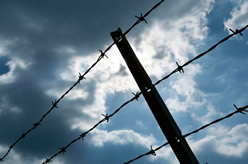Choose:
 908 feet 920 feet 5484 feet 5496 feet

2.
The perimeter of rectangle ABCD is 46 feet and AB = 15 feet. Find BD.

Choose:
 8 ft. 15 ft. 16 ft. 17 ft.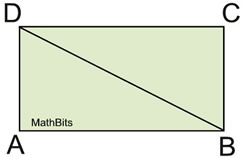3.
The circumference of a circle is 24π. Find the area of the circle.
Choose:
 576π 144π 36π 12π

4.
Given the diagram at the right.
a) Find the perimeter.
Choose:
 76" 69" 66" 59"

b) Find the area.
Choose:
 148 sq. in. 168 sq. in. 178 sq. in. 256 sq. in.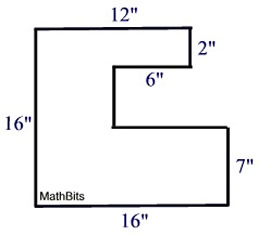Not drawn to scale.

5.
An arched window has dimensions as shown at the right. Consider the top of the window to be a semicircle.
a) How much weather stripping is needed to surround the outer edge of the window
(to nearest tenth of foot)?
Choose:
 18.0' 19.7' 24.4' 33.8'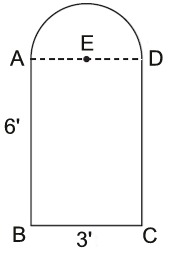b) How much plastic covering is needed to cover the window, following the outline of the window?
Choose:
 25.2 sq. ft. 42.0 sq. ft. 21.5 sq. ft. 36.7 sq.ft.

6.
The side of a regular hexagon measures 8 inches.
a) What is the perimeter of the hexagon?
Choose:
 40" 48" 56" 64"

b)
What is the area of the hexagon to nearest integer?

Choose:
 48 sq. in. 148 sq. in. 166 sq. in. 192 sq. in.

7.
Given the diagram at the right.
a)
Find the perimeter.

Choose:
 79 cm 94 cm 96 cm 111 cm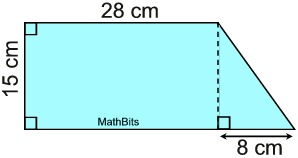b) Find the area.
Choose:
 480 sq. cm. 540 sq. cm 556 sq. cm. 564 sq. cm.

8.
The perimeter of a pentagon is 120".
a) If four sides measure 24.5", 18.2", 32.6", and 16.1", find the length of the fifth side.
Choose:
 36.6" 32.4" 29.6" 28.6"

b)
If the pentagon is regular, what is the length of its side?
Choose:
 12" 24" 28" 32"

9.
An isosceles trapezoid is labeled as shown. The perimeter of the trapezoid is 85 units. Find the value of x.
Choose:
 15 units 17½ units 19 units 19½ units10.
A large circular pizza has a circumference of 83 inches. Which of the perimeters listed could belong to the smallest box capable of holding a large pizza?
Choose:

 27" 54" 108" 135"